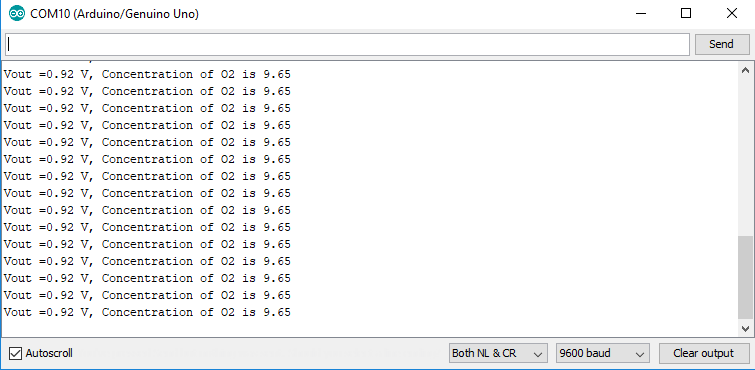# Arduino Grove - Gas Sensor(O₂)

Hello there

I bought a new Grove - Gas Sensor(O₂) and loaded the code from the Seed Wiki Website.

Unfortunately, the displayed values are totally wrong (see screenshot). Does any one of you have an idea why?

```[code]// Grove - Gas Sensor(O2) test code // Note: // 1. It need about about 5-10 minutes to preheat the sensor // 2. modify VRefer if needed```

const float VRefer = 3.3; // voltage of adc reference

void setup()
{
// put your setup code here, to run once:
Serial.begin(9600);
Serial.println(“Grove - Gas Sensor Test Code…”);
}

void loop()
{
// put your main code here, to run repeatedly:
float Vout =0;
Serial.print(“Vout =”);

``````Vout = readO2Vout();
Serial.print(Vout);
Serial.print(" V, Concentration of O2 is ");
delay(500);
``````

}

{
long sum = 0;
for(int i=0; i<32; i++)
{
}

``````sum >>= 5;

float MeasuredVout = sum * (VRefer / 1023.0);
return MeasuredVout;
``````

}

{
// Vout samples are with reference to 3.3V

``````//float Concentration = FmultiMap(MeasuredVout, VoutArray,O2ConArray, 6);
//when its output voltage is 2.0V,
float Concentration = MeasuredVout * 0.21 / 2.0;
float Concentration_Percentage=Concentration*100;
return Concentration_Percentage;
``````

}[/code]Hi there,

Please try this code include the calibration.

Thanks a lot it works !